Get ICSE Solutions for Class 10 Mathematics Chapter 12 Symmetry for ICSE Board Examinations on ICSESolutions.com. We provide step by step Solutions for ICSE Mathematics Class 10 Solutions Pdf. You can download the Class 10 Maths ICSE Textbook Solutions with Free PDF download option.

## Symmetry Class 10 Maths ICSE Solutions

### Figure Based Questions

Question 1. In each of the figure below, an additional line is indicated by dots. Observe, name the figure and state if the figure is symmetrical about the dotted line.Question 2. Every diameter of circle is an axis of the symmetry for the circle. Each of the figure below contain a circle or a part of a circle. State the number of axis of symmetry in each case and also indicate them.Question 3. Draw a line of symmetry of semi-circle.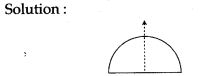Question 4. In the figure below are:
(a) an isosceles triangle;
(b) an equilateral triangle; and
(c) a scalene triangle state in each case if the triangle is a symmetrical figure. If a figure is symmetrical, state the number of axis of symmetry and indicate them by dotted line.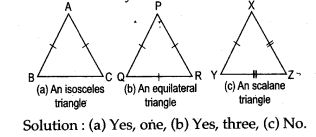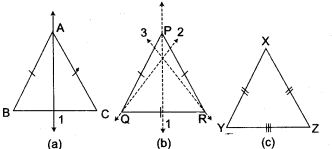Question 5. Draw a diagram and name the figure in the following:
(i) A quadrilateral having four lines of symmetry, two of which contain its diagonals.
(ii) A triangle with only one line of symmetry.
(iii) A quadrilateral having only one line of symmetry.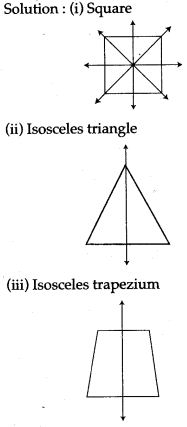Question 6. In each of the figure below, state the number of axes of symmetry, if any.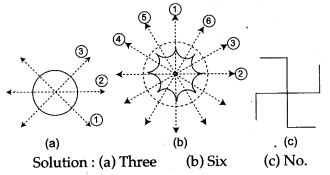Question 7. Draw an isosceles ΔABC, where BC = 3.5 cm, the base angles C and B = 75°. Use ruler and compass only. Draw all lines of symmetry of the triangle.Question 8. Construct a rectangle ABCD with AB = 5 cm and AD = 3 cm. Construct its lines of symmetry.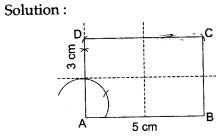Question 9. Draw an equilateral triangle each of whose side is 4 cm. Draw all its lines of symmetry.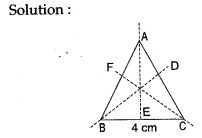Question 10. Draw two circles each of radius 2.5 cm touching each other. Construct the lines of symmetry of these circles.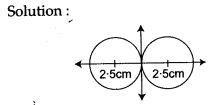Question 11. Use a ruler and compass only in this question.
(i) Construct the quadrilateral ABCD in which AB = 5 cm, BC = 7 cm and angle ABC = 120°, given that AC is its only line of symmetry.
(ii) Write down the geometrical name of the quadrilateral.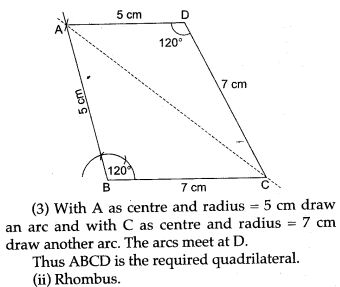Question 12. Part of geometrical figure is given in each of the diagrams below. Complete the figures, so that the line ‘m’, in each case, is the line of symmetry, of the completed figure. Recognizable free hand sketches, would be awarded full marks.Question 13. (i) Draw an equilateral triangle each of whose sides is 4 cm. Draw all its lines of symmetry.
(ii) Construct a ΔABC, in which AB = AC = 3 cm and BC = 2cm. Using a ruler and compasses only, draw the reflection of A’BC of ΔABC, in BC. Draw the lines of symmetry in the figure ABA’C.Question 14. A quadrilateral ABCD has exactly one axis of symmetry, which is not a diagonal. Show that the quadrilateral is an isosoeles trapezium.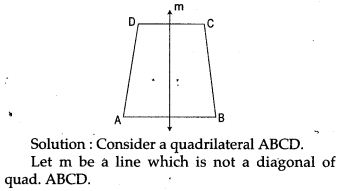Question 15. Part of a geometrical figure is given in each of the diagram below. Complete the figures so that both the X-axis and the Y-axis are lines of symmetry of the completed figure.Question 16. Part of a geometrical figure is given in each of the diagrams below. Complete the figures so. that the line AB in each case is a line of symmetry of the completed figure.
Give also the geometrical name for the completed figure. Recognizable free hand Sketches would be awarded full marks.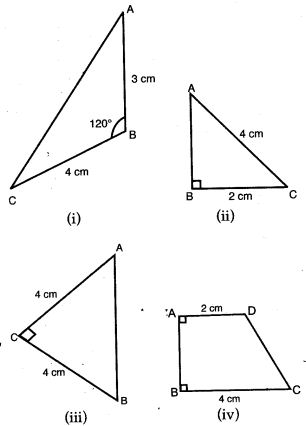For More Resources